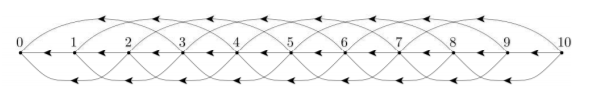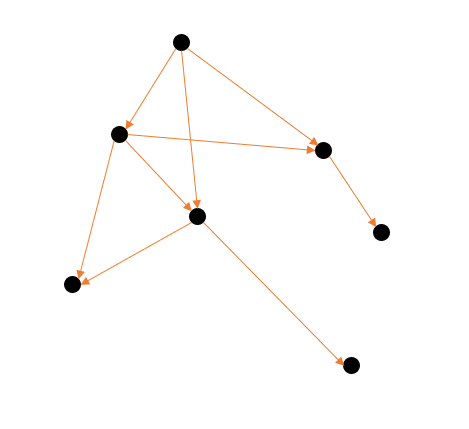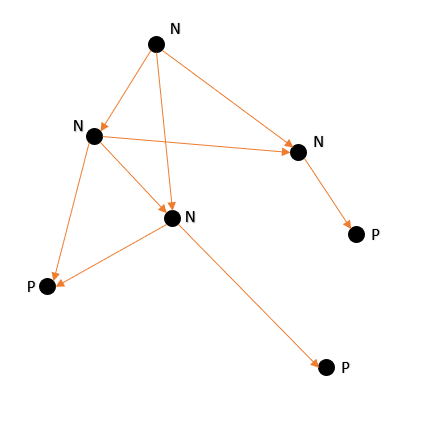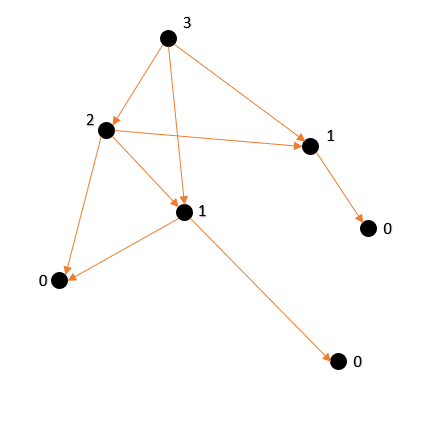×

Search anything:

# Sprague-Grundy Theorem and Game of Kayle

#### game theory List of Mathematical Algorithms sprague grundy theorem game of kayle AlgorithmsGet this book -> Problems on Array: For Interviews and Competitive Programming

Reading time: 25 minutes | Coding time: 10 minutes

Before we get to know what is Sprague-Grundy Theorem, we need to understand the significance of Sprague-Grundy functions. As we will see further, impartial games can be converted from games to graphs. I am going to provide one such visualisation example based on the fundamentals of Nim and later on extend the application to the Kayle's game, elaborating as much as possible and wherever possible.

A game consists of a graph G = (X, F) where:

• X is the set of all possible game positions
• F is a function that gives for each x ∈ X a subset of possible x’s to move to, called **followers**. If F(x) is empty, the position x is terminal.
• The start position is x0 ∈ X. So player 1 moves first from x0.
• Players alternate moves. At position x, the player chooses from y ∈ F(x).
• The player confronted with the empty set F(x) loses.

Now, a little heads up, we will be dealing with progressively bounded graphs, something like thisA progressively Bounded Graph

Now, what is Sprague Grundy Function? Sprague Grundy function of a graph G = (F,X) is a function g defined such that it follows:

\$\$g(x) = mex\ \{\ g(y)\ :\ y\ ⋹\ F(x)\ \}\$\$

In simpler words, g is a function which returns smallest non negative integer which is not in the given set. Also, I am going to abbreviate Sprague - Grundy to 'sg' from now on for the sake of simplicity.

### Algorithm

• Graphic Visualisation:

Notice that g(x) is defined recursively, so, let's assume some base terminal nodes.

Let terminal nodes g(x) = 0 and then set those nodes which follow only terminal nodes as g(x) = 1. The given below is our unlabelled graph.We will first start by letter each position as being either “N” or “P”. Label every node with no outgoing edges as P, since it is an endgame and by definition a P position. Every node pointing to a P node must be N, so go ahead and label those too. Now label all the positions that only go to N positions as P. You should end up with the following labels:Now, following our mex function's dynamics, we will label all P's as 0, then all the nodes only following the terminal nodes as 1 and then follow the mex{0,1,2,...} rule for labelling further.Notice that all vertices that have an SG value of 0 are P positions! All others are N. Sound familiar? So it looks like a good strategy on a game that can be represented in such a graph would be to move to a vertex with g(x) = 0.

So this is how you can manually manipulate the game in your favour accordingly by knowing the SG number from that game move!

• Mathematical Algorithm (The Main Sprague Grundy Theorem)

Now that we have understood what exactly is SG Function, it is safe to state SG Theorem now.

It states that the SG function for a sum of games on a graph is just the Nim sum of the SG functions of its components .

If gi is the Sprague-Grundy function of Gi , i = 1 . . .n, then G = G1+. . .+Gn has Sprague-Grundy function g(x1 . . .xn) = g1(x1) ⊕ gn(xn).

### Complexity

• For Grundy Numbers in Kayle's Game: Polynomial Function depending upon the kayle numbers required

### Implementations

Implementations of Grundy Number for The Game of Kayle is as follows:

• Python3

### Python3

``````
def SGsom(a, b):
x = 0
y = 2
while a != 0 or b != 0:
if (a%y == b%y):
w = 0
else:
w = 1
x += w*(y/2)
a -= a%y
b -= b%y
y *= 2
return x
def quickSort(array, left, right):
i = left
j = right
pivot = array[(left + right) / 2]
while (i <= j):
while (array[i] < pivot):
i += 1
while (array[j] > pivot):
j -= 1
if (i <= j):
array[i], array[j] = array[j], array[i]
i += 1
j -= 1
if (left < j):
quickSort(array, left, j)
if (i < right):
quickSort(array, i, right)
def main():
m = input("How Many Sprague Grundy Value do you want to know?")
n = m +1
main_arr = [0 for i in range(n)]
main_arr = 1
temp_arr = [0 for i in range(n)]
for i in range (2, n):
k = 0
l = 1
for j in range (((i-1)/2) + 1):
temp_arr[j] = SGsom(main_arr[j], main_arr[i-j-1])
for j in range (((i-2)/2) + 1):
temp_arr[j+(i+1)/2] = SGsom(main_arr[j], main_arr[i-j-2])
temp_arr[i] = i + 1
quickSort(temp_arr, 0, i-1)
while (k != 1):
if (temp_arr != 0):
k += 1
main_arr[i] = 0
elif (temp_arr[l] - temp_arr[l-1] <= 1):
l += 1
else:
k += 1
main_arr[i] = temp_arr[l-1] + 1
for i in range (m+1):
print (str(i)+"---"+str(main_arr[i]))
main()
``````

### Applications

We will be discussing the application of Sprague Grundy Theorem using a famous game of Kayle.

Kayles is played with a row of tokens, which represent bowling pins. The row may be of any length. The two players alternate; each player, on his or her turn, may remove either any one pin (a ball bowled directly at that pin), or two adjacent pins (a ball bowled to strike both). Under the normal play convention, a player loses when he or she has no legal move (that is, when all the pins are gone). The game can also be played using misère rules; in this case, the player who cannot move wins!Most players quickly discover that the first player has a guaranteed win in normal Kayles whenever the row length is greater than zero. This win can be achieved using a symmetry strategy. On his or her first move, the first player should move so that the row is broken into two sections of equal length. This restricts all future moves to one section or the other. Now, the first player merely imitates the second player's moves in the opposite row.

It is more interesting to ask what the nim-value is of a row of length n. This is often denoted Kn; it is a nimber, not a number. By the Sprague–Grundy theorem, Kn is the mex over all possible moves of the nim-sum of the nim-values of the two resulting sections.
One Such example is :

\$\$K_5 = mex\ \{\ K_0 + K_4\ ,\ K_1 + K_3\ ,\ K_2 + K_2\ ,\ K_0 + K_3\ ,\ K_1 + K_2 \}\$\$

because from a row of length 5, one can move to the positions

\$\$\ K_0 + K_4\ ,\ K_1 + K_3\ ,\ K_2 + K_2\ ,\ K_0 + K_3\ ,\ K_1 + K_2\ \$\$

### Questions

72
62
83
12

#### What is the octet number of Game of Kayles?

0.77
0.33
0.66
0.11